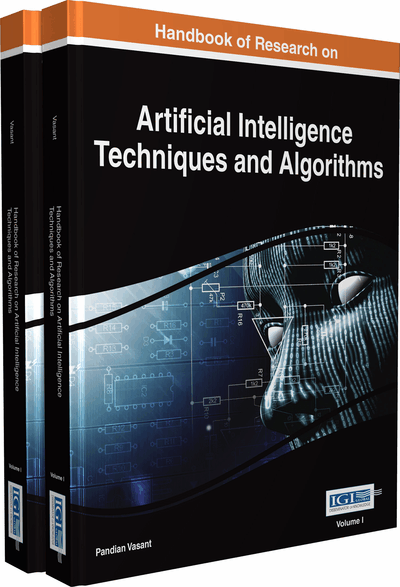# Robust Control and Synchronization of Chaotic Systems with Actuator Constraints

Kouamana Bousson (University of Beira Interior, Portugal) and Carlos Velosa (University of Beira Interior, Portugal)
Copyright: © 2015 |Pages: 43
DOI: 10.4018/978-1-4666-7258-1.ch001
OnDemand PDF Download:
Available
\$37.50
No Current Special Offers

## Abstract

This chapter proposes a robust control approach for the class of chaotic systems subject to magnitude and rate actuator constraints. The approach consists of decomposing the chaotic system into a linear part plus a nonlinear part to form an augmented system comprising the system itself and the integral of the output error. The resulting system is posteriorly seen as a linear system plus a bounded disturbance, and two robust controllers are applied: first, a controller based on a generalization of the Lyapunov function, then a Linear-Quadratic Regulator (LQR) with a prescribed degree of stability. Numerical simulations are performed to validate the approach applying it to the Lorenz chaotic system and to a chaotic aeroelastic system, and parameter uncertainties are also considered to prove its robustness. The results confirm the effectiveness of the approach, and the constraints are guaranteed as opposed to other control techniques which do not consider any kind of constraints.
Chapter Preview
Top

## 2. Background

Chaotic systems are deterministic nonlinear systems mostly with a relatively simple structure, which despite being governed by well-defined dynamical laws, exhibit an unpredictable motion and are characterized as being highly sensitive to the initial conditions and to parameter changes. The same chaotic system started at nearly initial states or with slightly different parameters produces trajectories in the phase-space which quickly become uncorrelated. Due to this, it is practically impossible to construct two identical chaotic systems in laboratory without an active control because the trajectories will never be equal or even similar.

## Key Terms in this Chapter

Trajectory/Orbit: A path (sequence of points) generated by a continuous or discrete system in its phase space.

Chaotic: Represents the type of motion that is highly sensitive to changes in the initial conditions, that is extremely sensitive to parameter changes, and that its behaviour is unpredictable for medium/long-term. Two trajectories initiated with slightly different initial conditions diverge exponentially over the time.

Phase Space: For dynamical systems governed by a set of first order differential equations is a space whose coordinates are the state variables or some components of the state vector. It is used to represent the system behaviour and a point in the phase space defines a potential state of the system.

Strange/Chaotic Attractor: Refers to an attractor set in the phase space with a fractal dimension in which the motions of the trajectories are chaotic.

Limit Cycle: An isolated closed trajectory in the phase space. The term isolated means that the neighbouring trajectories are not closed.

Lyapunov Exponents: Numbers that measure the rate of exponential attraction or separation over the time of two adjacent trajectories started with different initial conditions in the phase space. For a dynamical system with bounded trajectories, a positive Lyapunov exponent indicates the existence of a chaotic motion and if two or more exponents are positive the system is said to be hyperchaotic.

Underactuated Systems: Systems in which the number of control variables is less than the number of degrees of freedom.

Melnikov Function: A function that measures the distance between a stable manifold and an unstable manifold associated with a saddle point of a given Poincaré section resulting from a continuous flow. According to the Melnikov theory when the two manifolds intersect the function has a simple zero indicating the existence of chaos.

Poincaré Section: A sequence of points in the phase space generated by the intersection of a continuous flow with a given surface/section.

## Complete Chapter List

Search this Book:
Reset